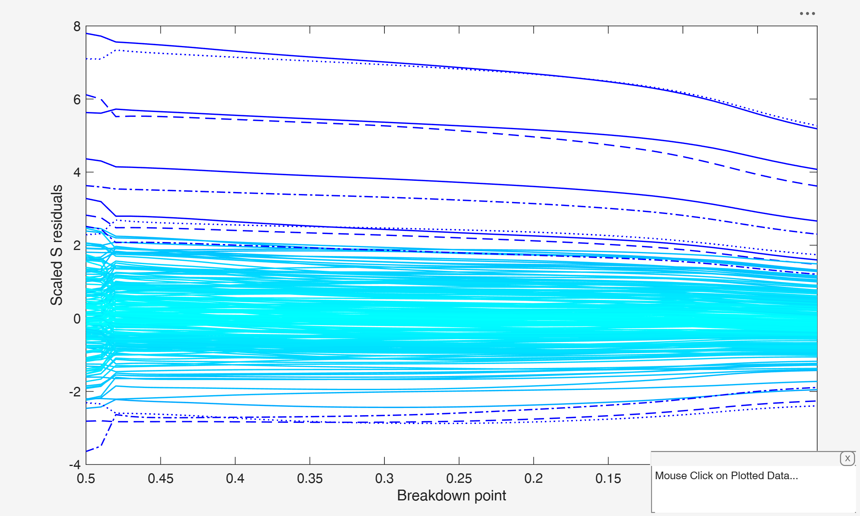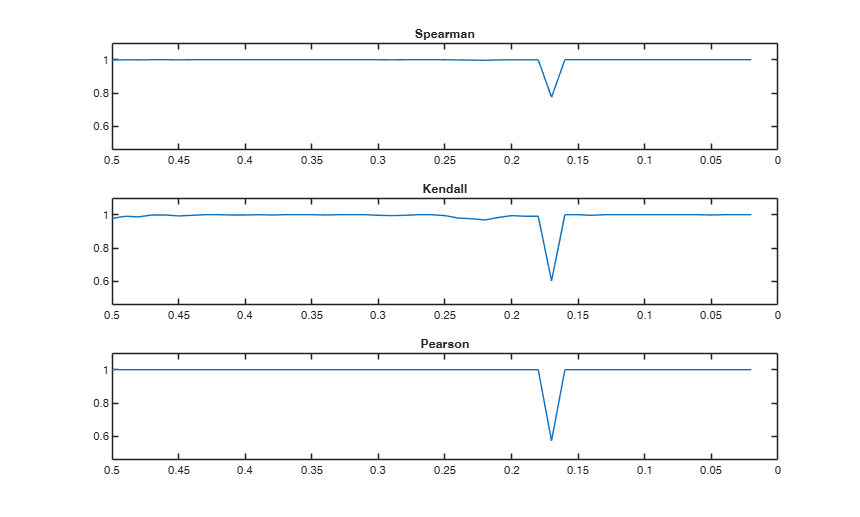# Sregeda

Sregeda computes S estimators in linear regression for a series of values of bdp

## Syntax

• out =Sregeda(y,X)example
• out =Sregeda(y,X,Name,Value)example
• [out , varargout]=Sregeda(___)example

## Description

 out =Sregeda(y, X) Sregeda with msg=0.

 out =Sregeda(y, X, Name, Value) Sreg with optional input arguments.

 [out , varargout] =Sregeda(___) Sreg with hyperbolic rho function.

## Examples

expand all

###Sregeda with msg=0.

Run this code to see the output shown in the help file

n=200;
p=3;
randn('state', 123456);
X=randn(n,p);
% Uncontaminated data
y=randn(n,1);
% Contaminated data
ycont=y;
ycont(1:5)=ycont(1:5)+6;
[out]=Sregeda(ycont,X,'msg',0);
resfwdplot(out)
ylabel('Scaled S residuals');### Sreg with optional input arguments.

Sreg with optimal rho function. Run this code to see the output shown in the help file

n=200;
p=3;
randn('state', 123456);
X=randn(n,p);
% Uncontaminated data
y=randn(n,1);
% Contaminated data
ycont=y;
ycont(1:5)=ycont(1:5)+6;
[out]=Sregeda(ycont,X,'rhofunc','optimal');

### Sreg with hyperbolic rho function.

Run this code to see the output shown in the help file

n=200;
p=3;
randn('state', 123456);
X=randn(n,p);
% Uncontaminated data
y=randn(n,1);
% Contaminated data
ycont=y;
ycont(1:5)=ycont(1:5)+6;
[out]=Sregeda(ycont,X,'rhofunc','hyperbolic');

## Related Examples

expand all

###Sreg on Stars data.

Run this code to see the Figure 2 of the article in the References

load('stars');
X=stars{:,1};
y=stars{:,2};
[out]=Sregeda(y,X,'rhofunc','bisquare');
standard.Color={'b'}
standard.xvalues=size(out.RES,1)-size(out.RES,2)+1:size(out.RES,1)
fground.Color={'r'}
resfwdplot(out,'standard',standard,'fground',fground)
ylabel('Scaled S residuals');
RHO = [];
for i=1:49
RHO(i,1) = corr(out.RES(:,i),out.RES(:,i+1),'type','Spearman');
RHO(i,2) = corr(out.RES(:,i),out.RES(:,i+1),'type','Kendall');
RHO(i,3) = corr(out.RES(:,i),out.RES(:,i+1),'type','Pearson');
end
minc = min(RHO);
maxc = max(RHO);
ylimits = [min(minc)*0.8,max(maxc)*1.1];
figure;
subplot(3,1,1);
plot(out.bdp(1:49),RHO(:,1)');
if strcmp(out.class,'Sregeda')
set(gca,'XDir','reverse','ylim',ylimits);
title('Spearman');
end
subplot(3,1,2);
plot(out.bdp(1:49),RHO(:,2)');
if strcmp(out.class,'Sregeda')
set(gca,'XDir','reverse','ylim',ylimits);
title('Kendall');
end
subplot(3,1,3);
plot(out.bdp(1:49),RHO(:,3)');
if strcmp(out.class,'Sregeda')
set(gca,'XDir','reverse','ylim',ylimits);
title('Pearson');
end
Total estimated time to complete S estimate:  0.86 seconds
Total estimated time to complete S estimate:  0.57 seconds
Total estimated time to complete S estimate:  0.58 seconds
Total estimated time to complete S estimate:  0.58 seconds
Total estimated time to complete S estimate:  0.52 seconds
Total estimated time to complete S estimate:  0.60 seconds
Total estimated time to complete S estimate:  0.55 seconds
Total estimated time to complete S estimate:  0.53 seconds
Total estimated time to complete S estimate:  0.51 seconds
Total estimated time to complete S estimate:  0.50 seconds
Total estimated time to complete S estimate:  0.53 seconds
Total estimated time to complete S estimate:  0.53 seconds
Total estimated time to complete S estimate:  0.58 seconds
Total estimated time to complete S estimate:  0.54 seconds
Total estimated time to complete S estimate:  0.54 seconds
Total estimated time to complete S estimate:  0.49 seconds
Total estimated time to complete S estimate:  0.51 seconds
Total estimated time to complete S estimate:  0.54 seconds
Total estimated time to complete S estimate:  0.47 seconds
Total estimated time to complete S estimate:  0.51 seconds
Total estimated time to complete S estimate:  0.45 seconds
Total estimated time to complete S estimate:  0.49 seconds
Total estimated time to complete S estimate:  0.44 seconds
Total estimated time to complete S estimate:  0.48 seconds
Total estimated time to complete S estimate:  0.47 seconds
Total estimated time to complete S estimate:  0.49 seconds
Total estimated time to complete S estimate:  0.50 seconds
Total estimated time to complete S estimate:  0.46 seconds
Total estimated time to complete S estimate:  0.42 seconds
Total estimated time to complete S estimate:  0.46 seconds
Total estimated time to complete S estimate:  0.47 seconds
Total estimated time to complete S estimate:  0.42 seconds
Total estimated time to complete S estimate:  0.47 seconds
Total estimated time to complete S estimate:  0.50 seconds
Total estimated time to complete S estimate:  0.60 seconds
Total estimated time to complete S estimate:  0.56 seconds
Total estimated time to complete S estimate:  0.60 seconds
Total estimated time to complete S estimate:  0.36 seconds
Total estimated time to complete S estimate:  0.33 seconds
Total estimated time to complete S estimate:  0.34 seconds
Total estimated time to complete S estimate:  0.30 seconds
Total estimated time to complete S estimate:  0.31 seconds
Total estimated time to complete S estimate:  0.28 seconds
Total estimated time to complete S estimate:  0.36 seconds
Total estimated time to complete S estimate:  0.30 seconds
Total estimated time to complete S estimate:  0.29 seconds
Total estimated time to complete S estimate:  0.30 seconds
Total estimated time to complete S estimate:  0.28 seconds
Total estimated time to complete S estimate:  0.28 seconds
Total estimated time to complete S estimate:  0.31 seconds

standard =

struct with fields:

Color: {'b'}

standard =

struct with fields:

Color: {'b'}
xvalues: [-2 -1 0 1 2 3 4 5 6 7 8 9 10 11 12 13 14 15 16 17 18 19 20 … ]

fground =

struct with fields:

Color: {'r'}

Warning: steps that you have chosen outside the range [m0 ... n]
are re-assigned to m0 or to n## Input Arguments

### y — Response variable. Vector.

A vector with n elements that contains the response variable. y can be either a row or a column vector.

Data Types: single| double

### X — Data matrix of explanatory variables (also called 'regressors') of dimension (n x p-1). Rows of X represent observations, and columns represent variables.

Missing values (NaN's) and infinite values (Inf's) are allowed, since observations (rows) with missing or infinite values will automatically be excluded from the computations.

Data Types: single| double

### Name-Value Pair Arguments

Specify optional comma-separated pairs of Name,Value arguments. Name is the argument name and Value is the corresponding value. Name must appear inside single quotes (' '). You can specify several name and value pair arguments in any order as  Name1,Value1,...,NameN,ValueN.

Example:  'bdp',[0.5 0.4 0.3 0.2 0.1] , 'bestr',10 , 'conflev',0.99 , 'intercept',false , 'minsctol',1e-7 , 'msg',0 , 'nocheck',true , 'nsamp',1000 , 'refsteps',10 , 'refstepsbestr',10 , 'reftol',1e-05 , 'reftolbestr',1e-10 , 'rhofunc','optimal' , 'rhofuncparam',5 , 'plots',0 

### bdp —breakdown point.scalar | vector.

It measures the fraction of outliers the algorithm should resist. In this case any value greater than 0 but smaller or equal than 0.5 will do fine.

The default for bdp is a sequence from 0.5 to 0.01 with step -0.01. The sequence is forced to be monotonically decreasing.

Example:  'bdp',[0.5 0.4 0.3 0.2 0.1] 

Data Types: double

### bestr —number of "best betas" to remember.scalar.

Scalar defining number of "best betas" to remember from the subsamples.

These will be later iterated until convergence (default=5).

Example:  'bestr',10 

Data Types: single | double

### conflev —Confidence level which is used to declare units as outliers.scalar.

Usually conflev=0.95, 0.975 0.99 (individual alpha) or 1-0.05/n, 1-0.025/n, 1-0.01/n (simultaneous alpha).

Default value is 0.975

Example:  'conflev',0.99 

Data Types: double

### intercept —Indicator for constant term.true (default) | false.

Indicator for the constant term (intercept) in the fit, specified as the comma-separated pair consisting of 'Intercept' and either true to include or false to remove the constant term from the model.

Example:  'intercept',false 

Data Types: boolean

### minsctol —tolerance for the iterative procedure for finding the minimum value of the scale.scalar.

Value of tolerance for the iterative procedure for finding the minimum value of the scale for each subset and each of the best subsets (It is used by subroutine minscale.m) The default value is 1e-7;

Example:  'minsctol',1e-7 

Data Types: single | double

### msg —Level of output to display.scalar.

It controls whether to display or not messages on the screen.

If msg==1 (default) messages are displayed on the screen about estimated time to compute the estimator and the warnings about 'MATLAB:rankDeficientMatrix', 'MATLAB:singularMatrix' and 'MATLAB:nearlySingularMatrix' are set to off else no message is displayed on the screen

Example:  'msg',0 

Data Types: single | double

### nocheck —Check input arguments.boolean.

If nocheck is equal to true no check is performed on matrix y and matrix X. Notice that y and X are left unchanged. In other words the additional column of ones for the intercept is not added.

As default nocheck=false.

Example:  'nocheck',true 

Data Types: boolean

### nsamp —Number of subsamples which will be extracted to find the robust estimator.scalar.

If nsamp=0 all subsets will be extracted.

They will be (n choose p).

If the number of all possible subset is <1000 the default is to extract all subsets otherwise just 1000.

Example:  'nsamp',1000 

Data Types: single | double

### refsteps —Number of refining iterations.scalar.

Number of refining iterationsin each subsample (default = 3).

refsteps = 0 means "raw-subsampling" without iterations.

Example:  'refsteps',10 

Data Types: single | double

### refstepsbestr —number of refining iterations for each best subset.scalar.

Scalar defining number of refining iterations for each best subset (default = 50).

Example:  'refstepsbestr',10 

Data Types: single | double

### reftol —tolerance for the refining steps.scalar.

The default value is 1e-6;

Example:  'reftol',1e-05 

Data Types: single | double

### reftolbestr —Tolerance for the refining steps.scalar.

Tolerance for the refining steps for each of the best subsets The default value is 1e-8;

Example:  'reftolbestr',1e-10 

Data Types: single | double

### rhofunc —rho function.string.

String which specifies the rho function which must be used to weight the residuals.

Possible values are 'bisquare' 'optimal' 'hyperbolic' 'hampel' 'mdpd'.

'bisquare' uses Tukey's $\rho$ and $\psi$ functions.

See TBrho and TBpsi.

'optimal' uses optimal $\rho$ and $\psi$ functions.

See OPTrho and OPTpsi.

'hyperbolic' uses hyperbolic $\rho$ and $\psi$ functions.

See HYPrho and HYPpsi.

'hampel' uses Hampel $\rho$ and $\psi$ functions.

See HArho and HApsi.

'mdpd' uses Minimum Density Power Divergence $\rho$ and $\psi$ functions.

See PDrho and PDpsi.

The default is bisquare

Example:  'rhofunc','optimal' 

Data Types: double

### rhofuncparam —Additional parameters for the specified rho function.scalar | vector.

For hyperbolic rho function it is possible to set up the value of k = sup CVC (the default value of k is 4.5).

For Hampel rho function it is possible to define parameters a, b and c (the default values are a=2, b=4, c=8)

Example:  'rhofuncparam',5 

Data Types: single | double

### plots —Plot on the screen.scalar.

If plots = 1, generates a plot with the robust residuals for each value of bdp. The confidence level used to draw the confidence bands for the residuals is given by the input option conflev. If conflev is not specified a nominal 0.975 confidence interval will be used.

Example:  'plots',0 

Data Types: single | double

## Output Arguments

### out — description Structure

A structure containing the following fields

Value Description
Beta

matrix containing the S estimator of regression coefficients for each value of bdp

Scale

vector containing the estimate of the scale (sigma) for each value of bdp. This is the value of the objective function

BS

p x 1 vector containing the units forming best subset associated with S estimate of regression coefficient.

RES

n x length(bdp) matrix containing the robust scaled residuals for each value of bdp

Weights

n x length(bdp) vector containing the estimates of the weights for each value of bdp

Outliers

Boolean matrix containing the list of the units declared as outliers for each value of bdp using confidence level specified in input scalar conflev

conflev

confidence level which has been used to declare outliers.

Singsub

Number of subsets wihtout full rank. Notice that out.singsub(bdp(jj)) > 0.1*(number of subsamples) produces a warning

rhofunc

string identifying the rho function which has been used

rhofuncparam

vector which contains the additional parameters for the specified rho function which have been used. For hyperbolic rho function the value of k =sup CVC. For Hampel rho function the parameters a, b and c. This field is present only if input argument 'rhofunc' is 'hyperbolic' or 'hampel'.

bdp

vector which contains the values of bdp which have been used

y

response vector y.

X

data matrix X.

class

'Sregeda'

### varargout —Indices of the subsamples extracted for computing the estimate (the so called elemental sets). C : matrix

Riani, M., Cerioli, A., Atkinson, A.C. and Perrotta, D. (2014), Monitoring Robust Regression, "Electronic Journal of Statistics", Vol. 8, pp. 646-677.

Maronna, R.A., Martin D. and Yohai V.J. (2006), "Robust Statistics, Theory and Methods", Wiley, New York.